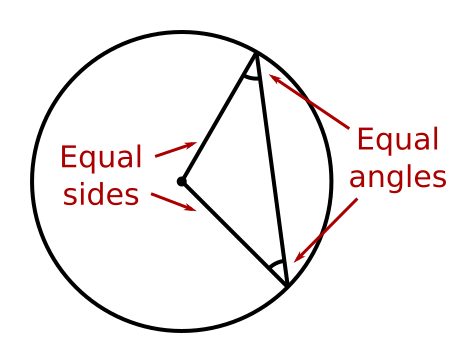# Two radii form an isosceles triangle

Martin McBride
2022-07-09

An isosceles triangle is a triangle that has two equal sides, like this:The two angles at the base of an isosceles triangle are also equal.

## Two radii of a circle

A triangle formed by two radii of a circle is an isosceles triangle:The radii form the two equal sides of the triangle. The third side is formed ny the chord that joins the ends of the two radii.

Since it is an isosceles triangle, it follows that the angles between the two radii and the chord that forms the third side of the triangle are also equal.

This theorem is covered in this video on circle theorems:

## Proof

A circle is a set of points that are all the same distance for the centre.

A radius is a line drawn from the centre to any point on the circle.

Since every point on the circle is the same distance from the centre, every radius is the same length no matter where it is drawn.

Two sides of the triangle are radii of the circle, and therefore have the same length. Any triangle with two equal sides is an isosceles triangle, by definition.